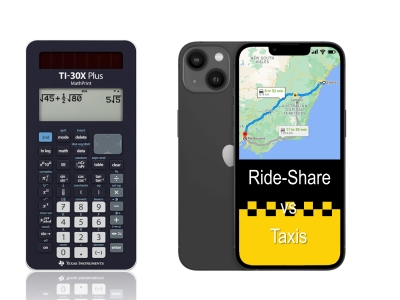# Activities

•• ##### AuthorAust Senior

20 Minutes

## Taxis v Rideshare#### Activity Overview

The aim of this activity is for students to use a table of values to compare two linear functions through the context of taxi and ride-share fares. After obtaining an estimate from the table of values, students then proceed to the algebraic solution.

#### Objectives

The aim of this activity is to provide a context and concept for solutions to simultaneous equations. Students start by exploring a table of values using their calculator and work towards solving the equations simultaneously (by hand).

#### Vocabulary

• Simultaneous Equations
• Equation
• Table of values